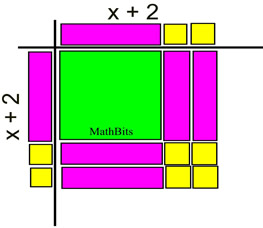Factoring Perfect Square Trinomials MathBitsNotebook.com Terms of Use   Contact Person: Donna RobertsWe have already discussed perfect square trinomials:
 Squaring a binomial creates a perfect square trinomial: (a + b)2 (a - b)2
 (a + b)2 = a2 + 2ab + b2 (a - b)2 = a2 - 2ab + b2

What we need to do now, is to "remember" these patterns
so that we can be on the look-out for them when factoring.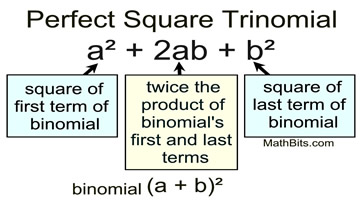Notice the Pattern of the middle term: The middle term is twice the product of the binomial's first and last terms. (a + b)² middle term +2ab (a - b)² middle term -2ab In (a - b), the last term is -b.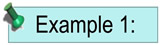Factor: x2 + 12x + 36 Solution: Does this fit the pattern of a perfect square trinomial? Yes. Both x2 and 36 are perfect squares, and 12x is twice the product of x and 6. Since all signs are positive, the pattern is (a + b)2 = a2 + 2ab + b2. Let a = x and b = 6. Answer:    (x + 6)2 or (x + 6)(x + 6)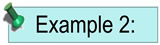Factor: 9a2 - 6a + 1 Solution: Does this fit the pattern of a perfect square trinomial? Yes. Both 9a2 and 1 are perfect squares, and 6a is twice the product of 3a and 1. Since the middle term is negative, the pattern is (a - b)2 = a2 - 2ab + b2. Let a = 3a and b = 1. Answer:   (3a - 1)2 or (3a - 1)(3a - 1)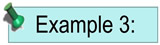Factor: (m + n)2 + 12(m + n) + 36 Solution: This is a sneaky one! Do NOT start by removing the parentheses. Look at the pattern, instead. . Does this fit the pattern of a perfect square trinomial? Yes. Both (m + n)2 and 36 are perfect squares, and 12(m + n) is twice the product of (m + n) and 6. Since the middle term is positive, the pattern is (a + b)2 = a2 + 2ab + b2. Let a = (m + n) and b = 6. Answer:  ((m + n) + 6)2 or (m + n + 6)2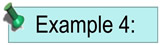Factor: x2 + 4x + 4 using Algebra Tiles
KEY: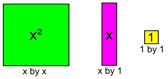See more about Algebra Tiles.
 Place the x2 tile, 4 x-tiles and 4 1-tiles in the grid.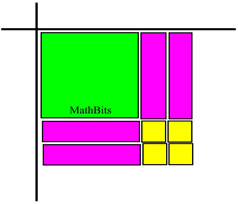Fill the outside sections of the grid with x-tiles and 1-tiles that complete the pattern.